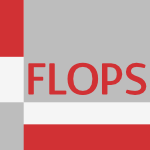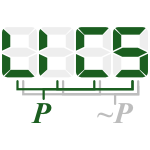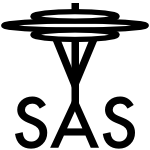`Used together with:`
type (22)
calculus (10)
term (6)
rewrit (4)
logic (4)

## Stem simpli\$ (all stems)

### 24 papers:FM-2014-LeinoM #automation #induction #proving #verification
Co-induction Simply — Automatic Co-inductive Proofs in a Program Verifier (KRML, MM), pp. 382–398.ICALP-v2-2013-Stirling #proving #λ-calculus
Proof Systems for Retracts in Simply Typed λ Calculus (CS), pp. 398–409.RTA-2012-Terui #complexity #evaluation #semantics #λ-calculus
Semantic Evaluation, Intersection Types and Complexity of Simply Typed λ Calculus (KT), pp. 323–338.TLCA-2011-BourreauS #game studies #semantics #λ-calculus
Game Semantics and Uniqueness of Type Inhabitance in the Simply-Typed λ-Calculus (PB, SS), pp. 61–75.VLDB-2006-Jhingran #enterprise
Enterprise Information Mashups: Integrating Information, Simply (AJ), pp. 3–4.CSL-2006-Aehlig #automaton #finite #infinity #semantics
A Finite Semantics of Simply-Typed λ Terms for Infinite Runs of Automata (KA), pp. 104–118.RTA-2005-AotoY #dependence #term rewriting
Dependency Pairs for Simply Typed Term Rewriting (TA, TY), pp. 120–134.ICALP-2004-Katsumata
A Generalisation of Pre-logical Predicates to Simply Typed Formal Systems (SyK), pp. 831–845.RTA-2003-AotoY #term rewriting #termination
Termination of Simply Typed Term Rewriting by Translation and Labelling (TA, TY), pp. 380–394.ESOP-2001-BossiERS #logic programming #scheduling #semantics #source code #termination
Semantics and Termination of Simply-Moded Logic Programs with Dynamic Scheduling (AB, SE, SR, JGS), pp. 402–416.FLOPS-2001-SatoSK #calculus
A Simply Typed Context Calculus with First-Class Environments (MS, TS, YK), pp. 359–374.CSL-2001-AbramskyL #modelling #λ-calculus
Fully Complete Minimal PER Models for the Simply Typed λ-Calculus (SA, ML), pp. 443–457.RTA-2001-Yamada #confluence #term rewriting #termination
Confluence and Termination of Simply Typed Term Rewriting Systems (TY), pp. 338–352.WRLA-2000-CirsteaK #calculus
The simply typed rewriting calculus (HC, CK), pp. 24–42.ICLP-1999-Bezem #logic programming #source code
Extensionality of Simply Typed Logic Programs (MB), pp. 395–410.TOOLS-USA-1998-Mellor98a #behaviour #how #modelling #question
Modeling Complex Behavior Simply or How Much is Too Much? (SJM), p. 444.LICS-1996-HillebrandK #on the #power of #λ-calculus
On the Expressive Power of Simply Typed and Let-Polymorphic λ Calculi (GGH, PCK), pp. 253–263.RTA-1996-FettigL #finite #higher-order #unification #λ-calculus
Unification of Higher-Order patterns in a Simply Typed λ-Calculus with Finite Products and terminal Type (RF, BL), pp. 347–361.TLCA-1995-Simpson #category theory #λ-calculus
Categorical completeness results for the simply-typed λ-calculus (AKS), pp. 414–427.SAS-1994-BanerjeeS #call-by #λ-calculus
Stackability in the Simply-Typed Call-by-Value λ Calculus (AB, DAS), pp. 131–146.LFP-1994-Cosmadakis #algebra #proving
Complete Proof Systems for Algebraic Simply-Typed Terms (SSC), pp. 220–226.STOC-1993-ChouK #2d #complexity
Some complexity issues on the simply connected regions of the two-dimensional plane (AWC, KIK), pp. 1–10.LICS-1992-LiguoroPS #λ-calculus
Retracts in simply typed λβη-calculus (Ud, AP, RS), pp. 461–469.ICLP-1991-Miller #logic programming #unification
Unification of Simply Typed Lamda-Terms as Logic Programming (DM), pp. 255–269.

Bibliography of Software Language Engineering in Generated Hypertext (BibSLEIGH) is created and maintained by Dr. Vadim Zaytsev.
Hosted as a part of SLEBOK on GitHub.